When naively raytracing (with triangle primitives), for n rays and m triangles there are mn intersection tests, as every ray must check for intersection with every triangle in the scene (ignoring triangle culling).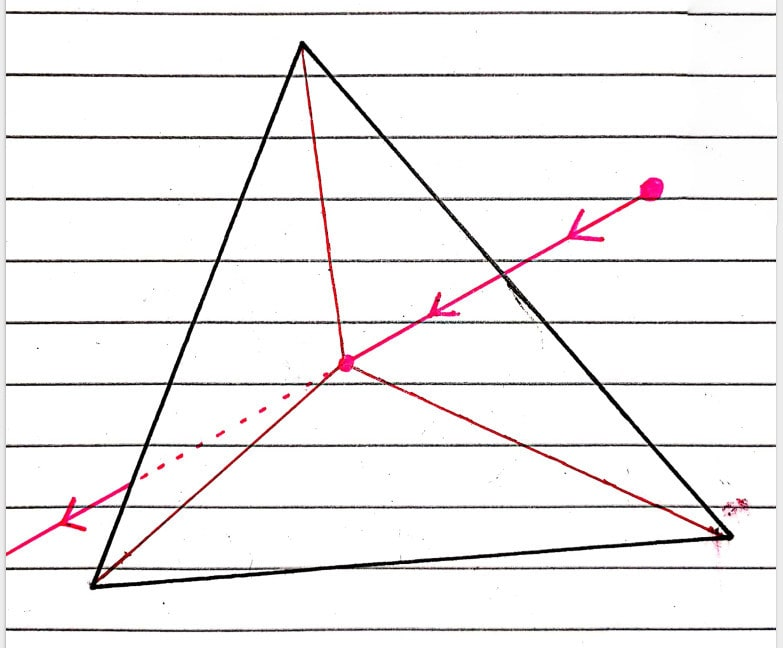This cost can be reduced with a spatial partitioning data structure, which groups primitives that are close together. This is useful because you can surround each group with some 'bounding volume' (BV), e.g. a sphere, and test intersections of the rays with the volume. If it doesn't intersect, then you are guaranteed that the ray does not intersect with any of the primitives in the group, as the volume surrounds the group. Thus you can completely ignore that group, saving on lots of useless computation.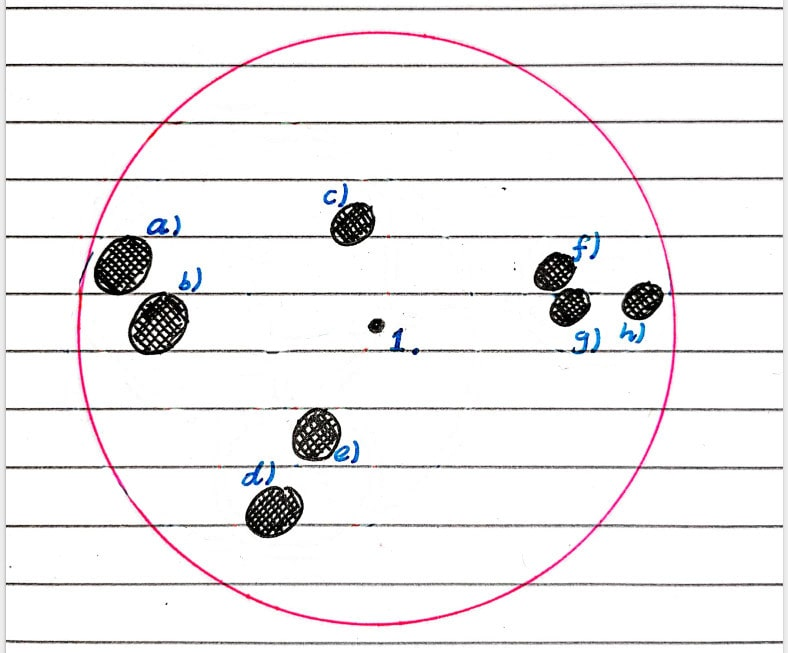If the BV is intersected with, then we need to check intersections with all the primitives in the group, as intersection with the BV only says that there 'may' be an intersection with a primitive in the group. It is very common for rays intersecting with the BV to completely miss all the primitives in the group. We can do this process multiple times, further partitioning groups of primitives into smaller and smaller groups, and creating a tree of bounding volumes. This is called a bounding volume hierarchy (BVH).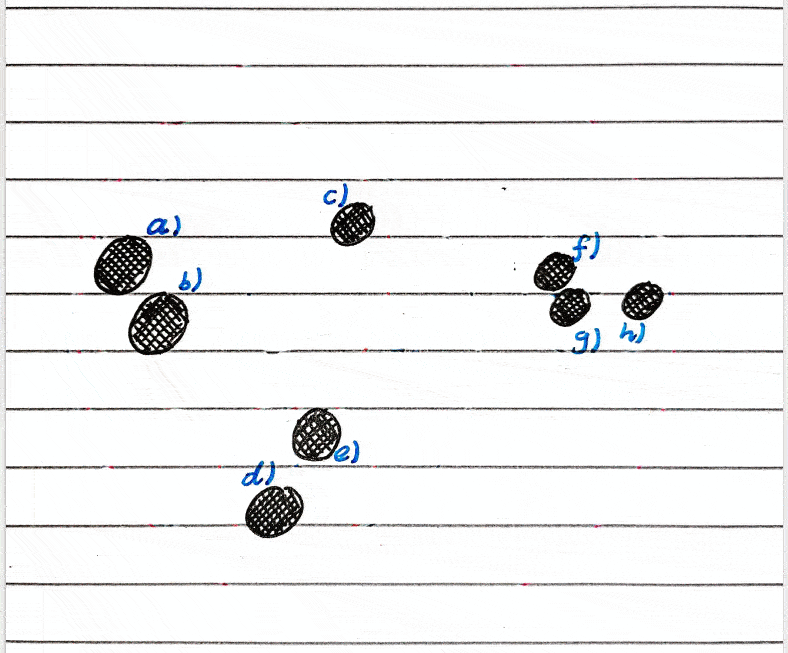For the BVH to have good performance, we ideally want every BV on a given level to be completely separate from each other, i.e. not intersecting one another. They should also conform as tightly as possible to the shape of the group, to minimise wasted intersection checks, and the tree should be as balanced as possible so that as many primitives as possible can be ignored during intersection testing. This maintains as closely as possible the O(nlogm) running time we're looking for with this data structure.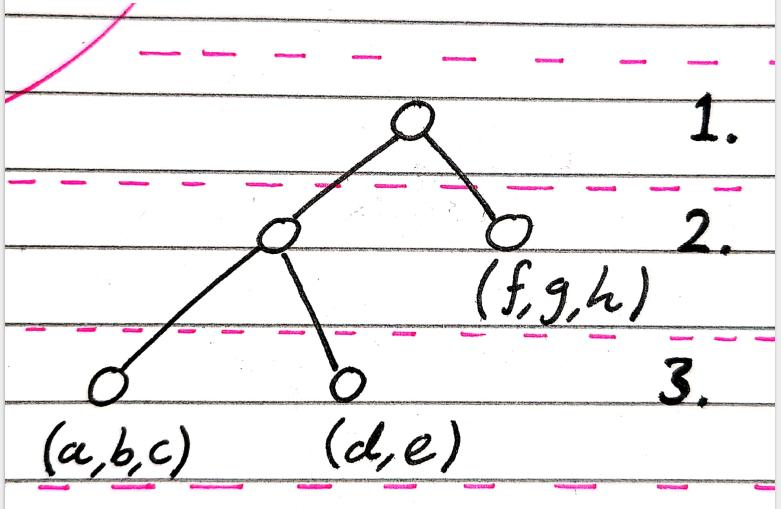The choice of the bounding volume is a tradeoff between conforming tightly to the shape of the group, and the speed of intersection tests with rays. We can use anything from spheres, which are incredibly cheap to test intersection with but risk unnecessarily huge volumes, and convex hulls, which fit the group perfectly but can be arbitrarily expensive to test intersections against. Personally I found spheres to be the fastest option, but I would recommend testing out axis-aligned bounding boxes if you have the time. Here is a good tutorial on ray-sphere intersection algorithms.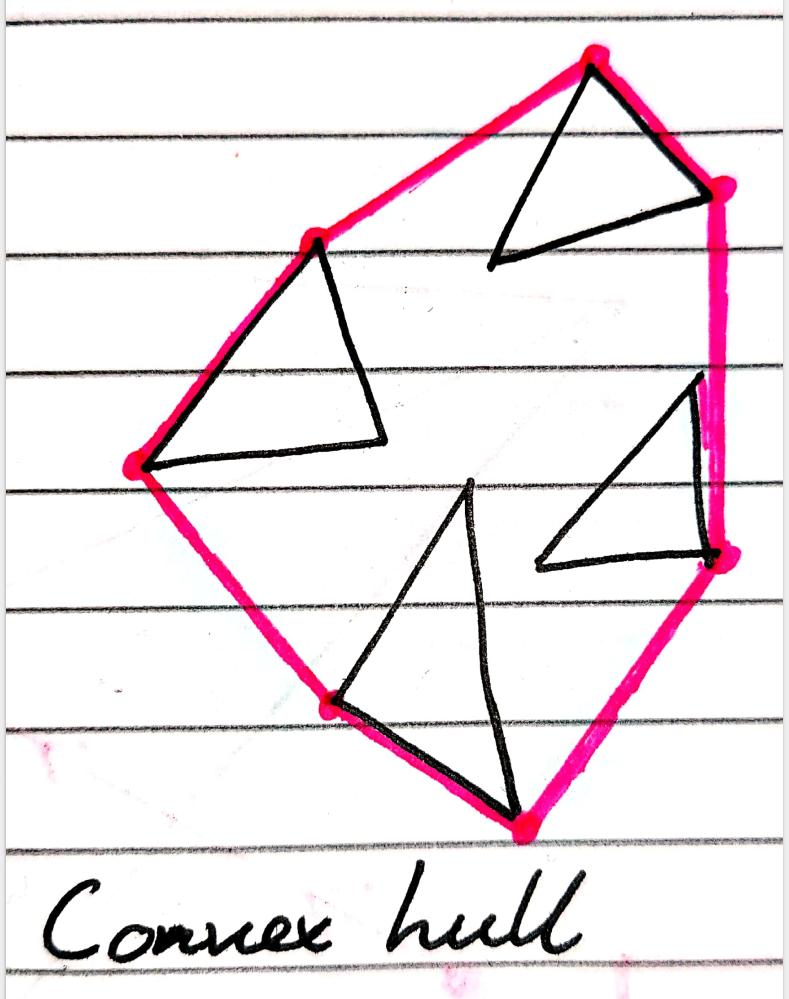I will be making a binary tree as these are by far the most common, but there’s nothing stopping you from making a tree of higher degree.

A non-ideal case of a sphere hierarchy, and a really non-ideal case.

##### Constructing the BVH

I’ll be starting with a 3D model imported from a ‘.obj’ file, a triangle mesh represented as an array of triangles. In my implementation I only use vertex information, no normals or UVs, as they’re unrelated to the BVH, and implementing them is it’s own task.

As we’re making a binary BVH, at every level we split the group of triangles into two groups. This splitting should be done so that each group is as spatially distinct as possible from the other. My quick and dirty solution is to calculate the variance in position of all the triangles in each dimension, relative to the centre of the BV of the group. In the process I also calculate the means position of the triangles. Then I find the dimension with the greatest variance as split along that axis, using the previously found mean position to get the mean along the splitting axis, defining the axis aligned plane that splits the triangles into two groups.

This approach is far from ideal, and has many cases with pretty awful BV choices for the subgroups. But it works, and improvement on it is left as an exercise for the reader :).

Once we have our two subgroups, we calculate the mean position of each subgroup, and find the minimum radius of each sphere that completely covers each subgroup, by iterating over each vertex in the subgroup and calculating the distance to the mean position. Once done, we have two new BVs for our subgroups, and we can recurse downwards again!

A major choice during the BVH construction is when to stop recursing down. We might naively keep going until we get a subgroup with a single element that can’t be split any further, but this only adds a rather unnecessary check when we should just be intersection testing against the triangle. With such small groups of triangles the benefits of a BVH can be quickly outweighed by it’s overhead.

This is a similar way of thinking to the way that the most efficient sorting algorithms are implemented, with quicksort at a high level for it’s asymptotic O(nlogn) running time, then when the sizes of the arrays to be sorted are reduced to a low enough number switching to insertion sort. Even though insertion sort has an O(n^2) running time, it can be quicker than quicksort on these small array slices due to quicksort’s overhead.

The approach I used was to just pick a minimum number of triangles that each group could have, and if a subgroup had that or below (due to splitting a group of size just above the minimum) then it wouldn’t recursively split and would instead store the triangles in it’s group.

##### Rendering with the BVH

As stated before, we test for intersection with the BV, and if that is true then we recursively test for intersection with it’s children.

The in-depth implementation of the BVH, along with a lot of extras, can be found here, along with a code repo here.